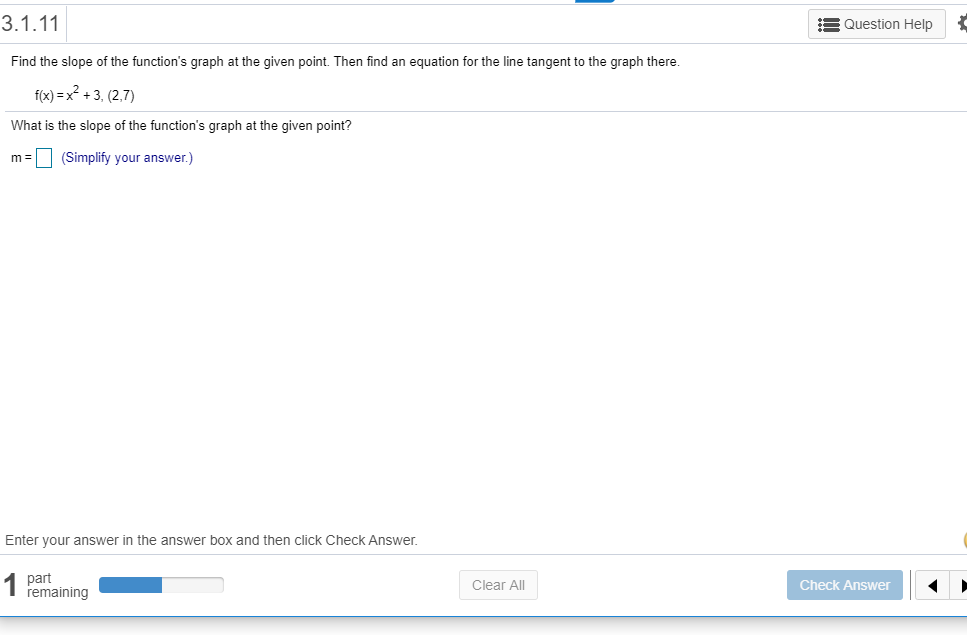# 3.1.11 Question Help Find the slope of the function's graph at the given point. Then find an equation for the line tangent to the graph there. f(x)= x2+3, (2,7) What is the slope of the function's graph at the given point? m (Simplify your answer.) Enter your answer in the answer box and then click Check Answer 1 part remaining Clear All Check Answer

Question

Can i get help with this problem step by step?help_outlineImage Transcriptionclose3.1.11 Question Help Find the slope of the function's graph at the given point. Then find an equation for the line tangent to the graph there. f(x)= x2+3, (2,7) What is the slope of the function's graph at the given point? m (Simplify your answer.) Enter your answer in the answer box and then click Check Answer 1 part remaining Clear All Check Answer fullscreen

## Expert Answer

### Want to see this answer and more?

Experts are waiting 24/7 to provide step-by-step solutions in as fast as 30 minutes!*

*Response times may vary by subject and question complexity. Median response time is 34 minutes for paid subscribers and may be longer for promotional offers.
Tagged in
Math
Calculus

### Derivative© 2021 bartleby. All Rights Reserved.